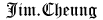# Professional Clojure

## Chapter 1 - Have a Beginner's Mind

In computer science, imperative programming is a programming paradigm that uses statements that change a program's state.

Value Oriented

Clojure promotes a style of programming commonly called "value-oriented programming."

``````(defn fib [n]
(cond
(= n 0) 0
(= n 1) 1
:else (+ (fib (- n 1))
(fib (- n 2)))))
``````

You can rewrite a function to leverage memorization to see a signicant improvement in the execution time:

``````(def memoized-fib
(memoize (fn [n]
(cond
(= n 0) 0
(= n 1) 1
:else (+ (fib (- n 1))
(fib (- n 2)))))))
``````

Thinking Recursively

A naive recursive defnition of a factorial in Clojure may look like the following:

``````(defn factorial [n]
(if (= n 1)
1
(* n (factorial (- n 1)))))
``````

tail recursive style (using `recur`):

``````(defn factorial2 [n]
(loop [count n acc 1]
(if (zero? count)
acc
(recur (dec count) (* acc count)))))
``````

`trampoline` and `letfn`:

``````(defn my-even? [n]
(letfn [(e? [n]
(if (= n 0)
true
#(o? (dec n))))
(o? [n]
(if (= n 0)
false
#(e? (dec n))))]
(trampoline e? n)))

(defn my-odd? [n]
(not (my-even? n)))
``````

higher order functions

``````(defn wrapInTransaction [f]
(do
(startTransaction)
(f)
(completeTransaction)))

(wrapInTransaction #(
(do